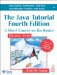# Operator Precedence

Table 65 lists the operators in the Java programming language. Operators higher in the table have higher precedence than do those lower in the table. Operators on the same line have the same precedence.

Table 65. Operator Precedence

Operator Category

Operators

Postfix operators

[] . (params) expr++ expr--

Unary operators

++expr --expr +expr -expr ~ !

Creation or cast

new (type)expr

Multiplicative

* / %

+ -

Shift

<< >> >>>

Relational

< > <= >= instanceof

Equality

== !=

Bitwise AND

&

Bitwise exclusive OR

^

Bitwise inclusive OR

|

Logical AND

&&

Logical OR

||

Conditional

?:

Assignment

= += -= *= /= %=

^= &= |= <<= >>= >>>=

When operators of equal precedence appear in the same expression, a rule must govern which is evaluated first. In the Java programming language, all binary operators except for the assignment operators are evaluated in left-to-right order. Assignment operators are evaluated in right-to-left order.The Java Tutorial: A Short Course on the Basics, 4th Edition
ISBN: 0321334205
EAN: 2147483647
Year: 2002
Pages: 125